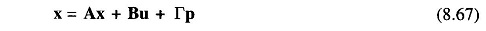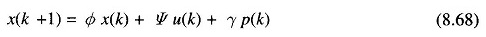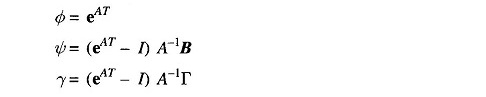## Discrete Time Control System:

The Discrete Time Control System is described by a set of linear differential equationswhere x, u, p are state, control and disturbance vectors respectively and A,B and Γ are constant matrices associated with the above vectors.

The discrete-time behavior of the continuous-time system is modelled by the system of first order linear difference equations:where x(k), u(k) and p(k) are the state, control and disturbance vectors and are specified at t = kT, k = 0, 1, 2, … etc. and T is the sampling period. Φ, Ψ and γ are the state, control and disturbance transition matrices and they are evaluated using the following relations.where A, B and Γ are the constant matrices associated with x, u, and p vectors in the corresponding continuous-time dynamic system. The matrix eAT can be evaluated using various well-documented approaches like Sylvestor’s expansion theorem, series expansion technique etc..

Scroll to Top